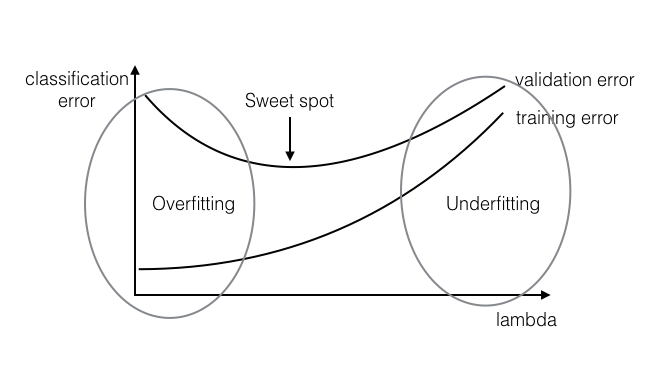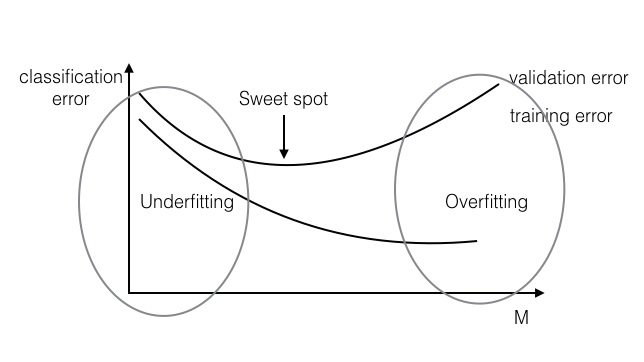## 11: Model Selection

### Make Sure Your Model is Optimally Tuned

Remember ERM $\min_{\mathbf{w}}\frac{1}{n}\sum_{i=1}^{n}\underset{Loss}{\underbrace{l_{(s)}(h_{\mathbf{w}}({\mathbf{x}_i}),y_{i})}}+\underset{Regularizer}{\underbrace{\lambda r(w)}}$ How should we set $\lambda$ (regulates the bias/variance)?

#### Overfitting and Underfitting

There are two equally problematic cases which can arise when learning a classifier on a data set: underfitting and overfitting, each of which relate to the degree to which the data in the training set is extrapolated to apply to unknown data:

Underfitting: The classifier learned on the training set is not expressive enough to even account for the data provided. In this case, both the training error and the test error will be high, as the classifier does not account for relevant information present in the training set.

Overfitting: The classifier learned on the training set is too specific, and cannot be used to accurately infer anything about unseen data. Although training error continues to decrease over time, test error will begin to increase again as the classifier begins to make decisions based on patterns which exist only in the training set and not in the broader distribution.Figure 1: overfitting and underfitting

#### Identify the Sweet Spot

Divide data into training and validation portions. Train your algorithm on the "training" split and evaluate it on the "validation" split, for various value of $\lambda$ (Typical values: 10-5 10-4 10-3 10-2 10-1 100 101 102 ...).

k-fold cross validation

Divide your training data into $k$ partitions. Train on $k-1$ of them and leave one out as validation set. Do this $k$ times (i.e. leave out every partition exactly once) and average the validation error across runs. This gives you a good estimate of the validation error (even with standard deviation). In the extreme case, you can have $k=n$, i.e. you only leave a single data point out (this is often referred to as LOOCV- Leave One Out Cross Validation). LOOCV is important if your data set is small and cannot afford to leave out many data points for evaluation .

Telescopic search

Do two searches: 1st, find the best order of magnitude for $\lambda$; 2nd, do a more fine-grained search around the best $\lambda$ found so far. For example, first you try $\lambda=0.01,0.1,1,10,100$. It turns out 10 is the best performing value. Then you try out $\lambda=5,10,15,20,25,...,95$ to test values "around" $10$.

#### Early Stopping

Stop your optimization after M (>= 0) number of gradient steps, even if optimization has not converged yet.Figure 2: Early stopping